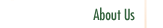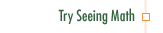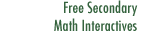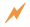Linear Functions

Linear Functions introduces algebra through the mathematically cohesive concept of functions and grounds it by modeling real-life situations.

Professional Development Goals

• Interpret the meaning and characteristics of linear functions in the context of real-world situations
• Identify ways in which multiple representations can express and enrich mathematical concepts
• Use piecewise functions to accentuate characteristics of linearity in the context of real-world modeling situationsClick to Get Seeing Math™ SecondaryCourse Listing Proportional Reasoning Linear Functions Transformations of Linear Functions Linear Equations Systems of Linear Equations Quadratic Functions Transformations of Quadratic Functions Quadratic Equations Data Analysis